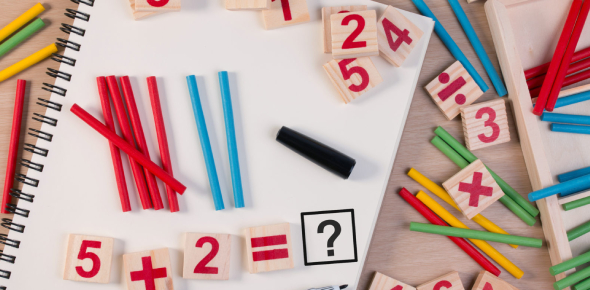# Basic Math Quiz For 7th Grade!

10 Questions | Total Attempts: 14704SettingsThis quiz has been designed for 7th-grade students to test basic mathematical questions based on division, multiplication, fractions, decimals, Percents, and ratios. Please read the questions carefully and answer. So, let's try out the quiz. All the best!

• 1.
What is 12*12?
• A.

114

• B.

144

• C.

14

• D.

24

• E.

36

• 2.
If a team won 10 games and lost 9 games, what is the ratio of games lost to games won?
• A.

10:9

• B.

10/9

• C.

9:1

• D.

9:10

• 3.
How would you show .25 in a fraction completely reduced?
• A.

25/100

• B.

2/10

• C.

25%

• D.

250/1000

• E.

1/4

• 4.
How would you show 1/5 as a decimal?
• A.

.2

• B.

200

• C.

2.00

• D.

.002

• 5.
In a bag full of small balls, 1/4 of these balls are green, 1/8 are blue, 1/12 are yellow and the remaining 26 white. How many balls are blue?
• A.

8 balls are blue

• B.

6 balls are blue

• C.

5 balls are blue

• D.

4 balls are blue

• 6.
In a school 50% of the students are younger than 10, 1/20 are 10 years old and 1/10 are older than 10 but younger than 12, the remaining 70 students are 12 years or older. How many students are 10 years old?
• A.

10 students are 10 years old

• B.

20 students are 10 years old

• C.

5 students are 10 years old

• D.

15 students are 10 years old

• 7.
What is the reciprocal of 3/4?
• A.

4/3

• B.

3/4

• C.

1/4

• D.

None of the above

• 8.
A bag has one orange marble, 4 white marbles, and 5 purple marbles. If you pick a marble 10 different times, what is the best prediction for picking a purple or white marble?
• A.

5

• B.

8

• C.

0

• D.

9

• 9.
Multiply. 2 x (-2)
• A.

4

• B.

2

• C.

-4

• D.

14

• 10.
Multiply. -2.9(-3)
• A.

-8.7

• B.

3.3

• C.

8.7

• D.

-3.3

Related TopicsBack to top